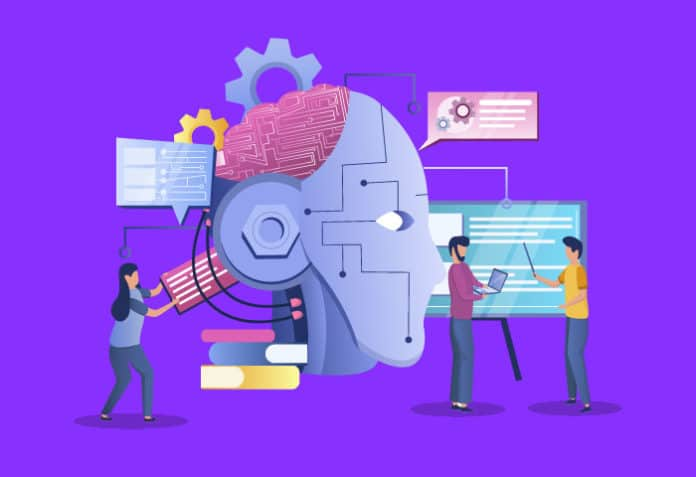Home Audience Developers Machine Learning: Classification Using Python

# Machine Learning: Classification Using Python

0
511In machine learning (ML), a set of data is analysed to predict a result. Python is considered one of the best programming language choices for ML. In this article, we will discuss machine learning with respect to classification using Python.

Let’s say you want to teach a child to differentiate between apples and oranges. There are various ways to do this. You could ask the child to touch both kinds of fruits so that they get familiar with the shape and the softness. You could also show her multiple examples of apples and oranges, so that they can visually spot the differences. The technological equivalent of this process is known as machine learning.

Machine learning teaches computers to solve a particular problem, and to get better at it through experience. The example discussed here is a classification problem, where the machine is given various labelled examples, and is expected to label an unlabelled sample using the knowledge it acquired from the labelled samples. A machine learning problem can also take the form of regression, where it is expected to predict a real-valued solution to a given problem based on known samples and their solutions. Classification and regression are broadly termed as supervised learning. Machine learning can also be unsupervised, where the machine identifies patterns in unlabelled data, and forms clusters of samples with similar patterns. Another form of machine learning is reinforcement learning, where the machine learns from its environment by making mistakes.

Classification
Classification is the process of predicting the label of a given set of points based on the information obtained from known points. The class, or label, associated with a data set could be binary or multiple in nature. As an example, if we have to label the sentiment associated with a sentence, we could label it as positive, negative or neutral. On the other hand, problems where we have to predict whether a fruit is an apple or an orange will have binary labels. Table 1 gives a sample data set for a classification problem.

In this table, the value of the last column, i.e., loan approved, is expected to be predicted based on the other variables. In the subsequent sections, we will learn how to train and evaluate a classifier using Python.

 Age Credit rating Job Property owned Load approval 35 good yes yes yes 32 poor yes no no 22 fair no no no 42 good yes no yes

Table 1

Training and evaluating a classifier
In order to train a classifier, we need to have a data set containing labelled examples. Though the process of cleaning the data is not covered in this section, it is recommended that you read about various data preprocessing and cleaning techniques before feeding your data set to a classifier. In order to process the data set in Python, we will import the pandas package and the data frame structure. You may then choose from a variety of classification algorithms such as decision tree, support vector classifier, random forest, XG boost, ADA boost, etc. We will look at the random forest classifier, which is an ensemble classifier formed using multiple decision trees.

```from sklearn.ensemble import RandomForestClassifier
from sklearn import metrics

classifier = RandomForestClassifier()

#creating a train-test split with a proportion of 70:30
X_train, X_test, y_train, y_test = train_test_split(X, y, test_size=0.33)

classifier.fit(X_train, y_train) #train the classifier on the training set

y_pred = classifier.predict(X_test) #evaluate the classifier on unknown data

print(“Accuracy: “, metrics.accuracy_score(y_test, y_pred)) #compare the predictions with the actual values in the test set```

Although this program uses accuracy as the performance metric, a combination of metrics should be used, as accuracy tends to generate non-representative results when the test set is imbalanced. For instance, we will get a high accuracy if the model gives the same prediction for every record and the data set that is used to test the model is imbalanced, i.e., most of the records in the data set have the same class that the model predicted.

Tuning a classifier
Tuning refers to the process of modifying the values of the hyperparameters of a model in order to improve its performance. A hyperparameter is a parameter whose value can be changed to improve the learning process of the algorithm.

The following code depicts random search hyperparameter tuning. In this, we define a search space from which the algorithm will pick different values, and choose the one that produces the best results:

```from sklearn.model_selection import RandomizedSearchCV

#define the search space
min_samples_split = [2, 5, 10]
min_samples_leaf = [1, 2, 4]
grid = {‘min_samples_split’ : min_samples_split, ‘min_samples_leaf’ : min_samples_leaf}

classifier = RandomizedSearchCV(classifier, grid, n_iter = 100)

#n_iter represents the number of samples to extract from the search space
#result.best_score and result.best_params_ can be used to obtain the best performance of the model, and the best values of the parameters

classifier.fit(X_train, y_train)```

Voting classifier
You can also use multiple classifiers and their predictions to create a model that will give a single prediction based on the individual predictions. This process (in which only the number of classifiers that voted for each prediction is considered) is called hard voting. Soft voting is a process in which each classifier generates a probability of a given record belonging to a particular class, and the voting classifier generates as its prediction, the class that obtained the maximum probability.

A code snippet for creating a soft voting classifier is given below:

```soft_voting_clf = VotingClassifier(